# Force And Laws Of Motion Class 9 CBSE Notes - Chapter 9

According to the CBSE Syllabus 2023-24, this chapter has been renumbered as Chapter 8.

## Introduction to Force

A force is an effort that changes the state of an object at rest or at motion. It can change an object’s direction and velocity. Force can also change the shape of an object.

### Effects of Force

Some effects of force include the following:

• Force moves stationary objects
• Force stops objects from moving
• Force changes the shape of a body
• Force changes the direction of motion

Push is defined as an action of force which causes an object to move from its place. The following are examples of push:

• Opening and closing the door
• Pushing the table
• Pushing a car
• Pushing of thumb pins
• Walking

Pull is defined as an action to make something move by either tugging or dragging. The following are examples of pull:

• Plucking the string of a guitar
• Pulling ropes while playing tug of war
• Opening the drawer
• Pulling the window curtain
• Opening and closing the doors

To know more about Force, visit here.

### Chapter Summary### Balanced and Unbalanced Forces

When balanced forces are applied to an object, there will be no net effective force acting on the object. Balanced forces do not cause a change in motion.

Unbalanced forces acting on an object change its speed and/or direction of motion. It moves in the direction of the force with the highest magnitude.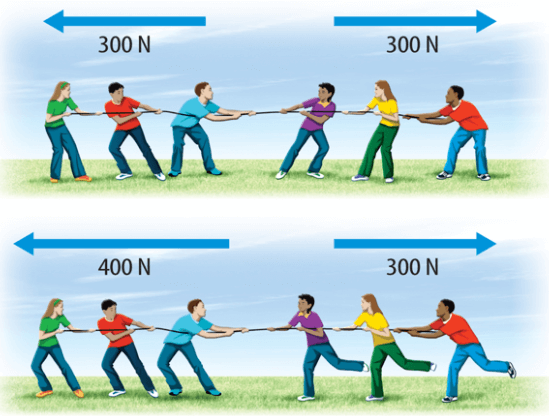### Net Force

When multiple forces act on a body, they can be resolved into one component known as the net force acting on the object. The net force decides the direction of motion.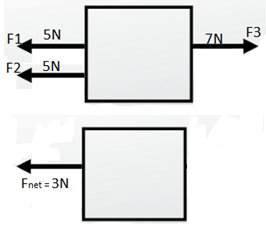To know more about Types of Forces and Their Nature, visit here.

## Frictional Force

The force that opposes relative motion is called friction. It arises between the surfaces in contact.

Example: When we try to push a table and it does not move is because it is balanced by the frictional force.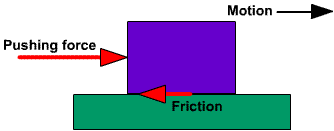### First Law of Motion

A body continues to be in the state of rest or uniform motion in a straight line unless acted upon by an external unbalanced force. The First Law is also called the Law of Inertia.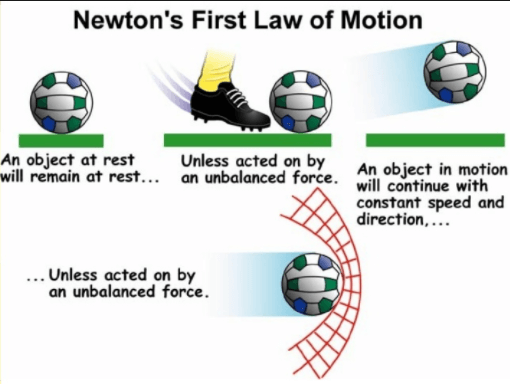To know more about the First Law of Motion, visit here.

### Inertia

Basically, all objects have a tendency to resist the change in the state of motion or rest. This tendency is called inertia. All bodies do not have the same inertia. Inertia depends on the mass of a body. The mass of an object is the measure of its inertia.

More the mass more the inertia and vice versa.

#### Inertia of Rest

An object stays at rest, and it remains at rest until an external force affects it. Example: When a car accelerates, passengers may feel as though their bodies are moving backwards. In reality, inertia is making their bodies stay in place as the car moves forward.

#### Inertia of Motion

An object will continue to be in motion until a force acts on it. Example: A hockey puck will continue to slide across the ice until acted upon by an outside force.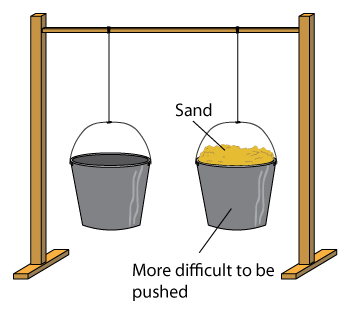## Second Law of Motion

In order to understand the Second Law, we need to first understand momentum.

### Momentum

Impacts produced by objects depend on their mass and velocity. The momentum of an object is defined as the product of its mass and velocity. p = mv. Vector quantity has direction and magnitude. An example of momentum is a baseball flying through the air and a bullet fired from a gun.

### Second Law of Motion

The rate of change of momentum of an object is directly proportional to the applied unbalanced force in the direction of the force.

$$\begin{array}{l}\frac{\Delta p}{t}\alpha \frac{m(v-u)}{t}\end{array}$$

Here, a [ = (v – u)/t ] is the acceleration, which is the rate of change in velocity.

$$\begin{array}{l}\frac{\Delta p}{t}\alpha ma\end{array}$$
$$\begin{array}{l}F \alpha ma\end{array}$$

F = kma

For 1 unit of force on 1 kg mass with the acceleration of 1m/s2, the value of k = 1.

Therefore, F = ma.

To know more about the Second Law of Motion, visit here.

## Conservation of Momentum

### Concept of System

• The part of the universe chosen for analysis is called a system.
• Everything outside the system is called an environment.
• For example, a car moving with constant velocity can be considered a system. All the forces within the car are internal forces, and all forces acting on the car from the environment are external forces like friction.

### Conservation of Momentum

• The total momentum of an isolated system is conserved.
• Isolated system net external force on the system is zero.
• Example: Collision of 2 balls, A and B.

From Newtons 3rd law F_{AB} = -F_{BA}

$$\begin{array}{l}m_{A}\frac{V_{a}-U_{a}}{t} = m_{B}\frac{V_{b}-U_{b}}{t}\end{array}$$
$$\begin{array}{l}m_{A}U_{A} + m_{B}U_{B} = m_{A}V_{A} + m_{B}V_{B}\end{array}$$

To know more about the Conservation of Momentum, visit here.

## Third Law of Motion

Newton’s 3rd law states that every action has an equal and opposite reaction. Action and reaction forces are equal, opposite and acting on different bodies.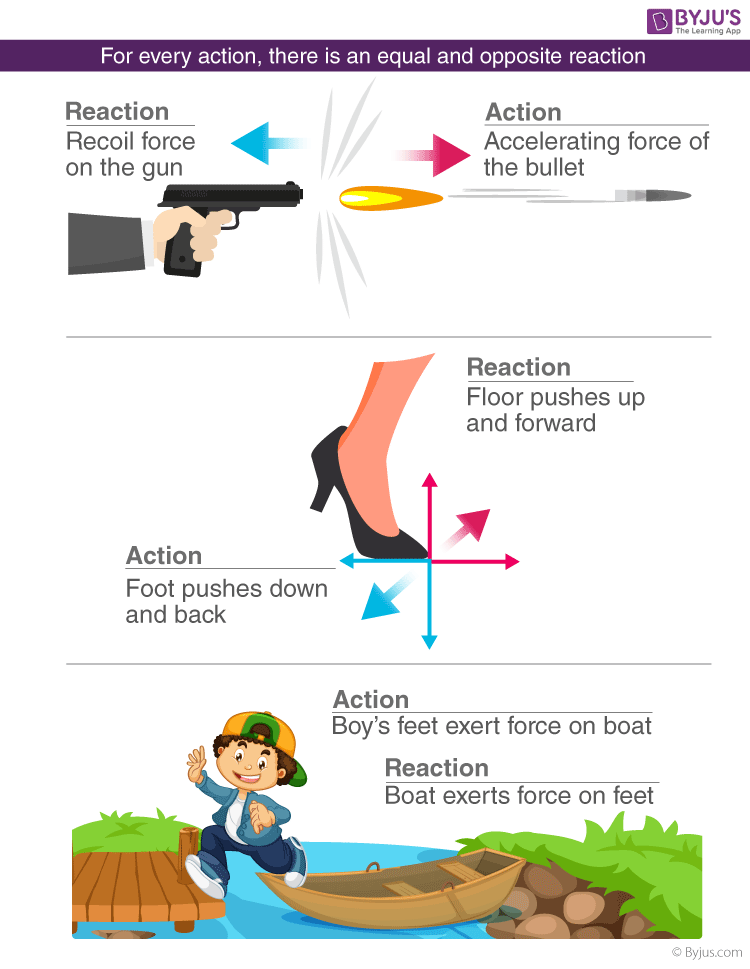To know more about the Third Law of Motion, visit here.

### Inertial and Non-Inertial Frames

• A non-inertial frame of reference is a frame of reference in which Newton’s laws of motion do not hold. A non-inertial reference frame is a frame of reference that is undergoing acceleration with respect to an inertial frame. An accelerometer at rest in a non-inertial frame will, in general, detect a non-zero acceleration.
• A frame of reference where Newton’s Laws hold is known as an inertial frame of reference.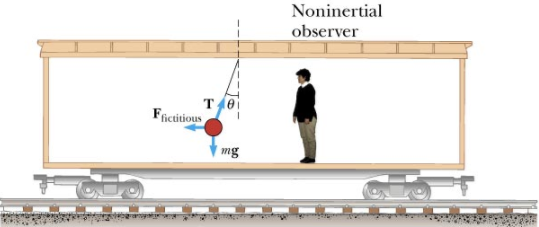#### For more information on understanding the Laws of Motion, watch the below video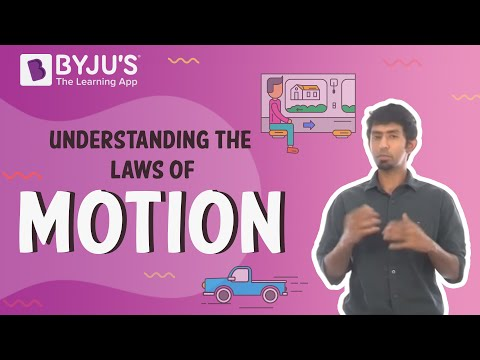To know more about the Laws of Motion, visit here.

## Frequently Asked Questions on CBSE Class 9 Science Notes Chapter 9 Force and Laws of Motion

Q1

### What is ‘Force’?

A force is a push or a pull upon an object resulting from the object’s interaction with another object.

Q2

### How many laws of motion are there?

There are totally 3 laws of motion.

Q3

### What is an ‘unbalanced force’?

When the forces applied to an object in opposite directions are not equal in size, they are known to be unbalanced forces.